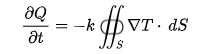# Fourier's Law

## What Is Fourier’s Law?

Fourier’s law states that the negative gradient of temperature and the time rate of heat transfer is proportional to the area at right angles of that gradient through which the heat flows. Fourier’s law is the other name of the law of heat conduction.

Newton’s law of cooling and Ohm’s law are a discrete and electrical analog of Fourier’s law.

## Differential Form Of Fourier’s Law

Fourier’s law differential form is as follows:

 $$\begin{array}{l}q=-k\bigtriangledown T\end{array}$$

Where,

• q is the local heat flux density in W.m2
• k is the conductivity of the material in W.m-1.K-1
• ▽T is the temperature gradient in K.m-1

In one-dimensional form:

 $$\begin{array}{l}q_{x}=-k\frac{\mathrm{d} T}{\mathrm{d} x}\end{array}$$

Integral formWhere,

• $$\begin{array}{l}\frac{\partial Q}{\partial t}\end{array}$$
is the amount of heat transferred per unit time
• dS is the surface area element

When the same equation is given in the differential form, which is the basis of heat equation derivation:

 $$\begin{array}{l}\frac{Q}{\bigtriangleup t}=-kA(\frac{\bigtriangleup T}{\bigtriangleup x})\end{array}$$

Where,

• A is the area of the cross-sectional surface
• ΔT is the temperature difference between the endpoints
• Δx is the distance between two ends

### Fourier’s law in terms of conductance

 $$\begin{array}{l}\frac{\bigtriangleup Q}{\bigtriangleup t}=UA(-\bigtriangleup T)\end{array}$$

Where,

• U is the conductance

### Fourier’s Law Derivation

The derivation of Fourier’s law was explained with the help of an experiment which explained the Rate of heat transfer through a plane layer is proportional to the temperature gradient across the layer and heat transfer area.

$$\begin{array}{l}Rate of heat conduction\propto \frac{(area)(temperature\;difference)}{thickness}\end{array}$$

Let T1 and T2 be the temperature difference across a small distance Δx of area A. k is the conductivity of the material. Therefore, in one dimensional, the following is the equation used:

$$\begin{array}{l}Q_{cond}=kA\frac{T_{1}-T_{2}}{\Delta x}=-kA\frac{\Delta T}{\Delta x}\end{array}$$

When Δx → 0, following is the equation in a reduced form to a differential form:

$$\begin{array}{l}Q_{cond}=-kA\frac{\Delta T}{\Delta x}\end{array}$$

The three-dimensional form the Fourier’s law is given as:

$$\begin{array}{l}\vec{q}=-k\bigtriangledown T\end{array}$$

Related Physics laws:

## Frequently Asked Questions – FAQs

### State Fourier’s law.

Fourier’s law states that the negative gradient of temperature and the time rate of heat transfer is proportional to the area at right angles of that gradient through which the heat flows.

### Give the diffrential form of the Fourier law.

$$\begin{array}{l}q=-k\bigtriangledown T\end{array}$$

### Give the three-dimensional form the Fourier’s law.

The three-dimensional form the Fourier’s law:
$$\begin{array}{l}\vec{q}=-k\bigtriangledown T\end{array}$$

### What is Fourier’s law also known as?

The Fourier’s law also known as the law of heat conduction.

### State true or false: Newton’s law of cooling is a discrete and electrical analog of Fourier’s law.

True.

Test Your Knowledge On Fouriers Law!